# How to calculate MOVING AVERAGE in a Pandas DataFrame?

In this article, we will be looking at how to calculate the moving average in a pandas DataFrame. Moving Average is calculating the average of data over a period of time. The moving average is also known as the rolling mean and is calculated by averaging data of the time series within k periods of time.

### There are three types of moving averages:

• Simple Moving Average (SMA)
• Exponential Moving Average (EMA)
• Cumulative Moving Average(CMA)

The link to the data used is RELIANCE.NS_

## Simple Moving Average (SMA)

A simple moving average tells us the unweighted mean of the previous K data points. The more the value of K the more smooth is the curve, but increasing K decreases accuracy. If the data points are p1,  p2, . . . , pn then we calculate the simple moving average.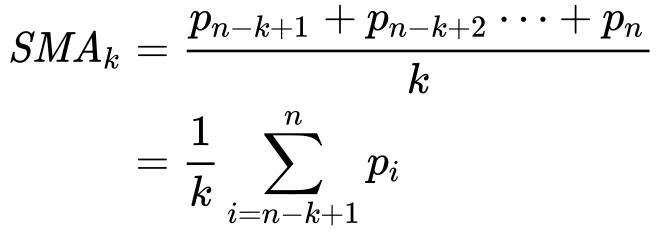In Python, we can calculate the moving average using .rolling() method. This method provides rolling windows over the data, and we can use the mean function over these windows to calculate moving averages. The size of the window is passed as a parameter in the function .rolling(window).

Now let’s see an example of how to calculate a simple rolling mean over a period of 30 days.

## Python3

 `# importing Libraries`   `# importing pandas as pd` `import` `pandas as pd`   `# importing numpy as np` `# for Mathematical calculations` `import` `numpy as np`   `# importing pyplot from matplotlib as plt` `# for plotting graphs` `import` `matplotlib.pyplot as plt` `plt.style.use(``'default'``)` `%``matplotlib inline`

### Step 2: Importing Data

To import data we will use pandas  .read_csv() function.

## Python3

 `# importing time-series data` `reliance ``=` `pd.read_csv(``'RELIANCE.NS.csv'``, index_col``=``'Date'``,` `                       ``parse_dates``=``True``)`   `# Printing dataFrame` `reliance.head()`

Output: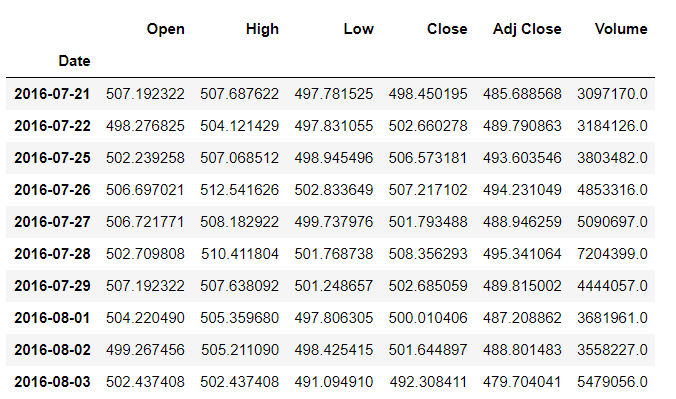### Step 3: Calculating Simple Moving Average

To calculate SMA in Python we will use Pandas dataframe.rolling() function that helps us to make calculations on a rolling window. On the rolling window, we will use .mean() function to calculate the mean of each window.

Syntax: DataFrame.rolling(window, min_periods=None, center=False, win_type=None, on=None, axis=0).mean()

Parameters :

• window : Size of the window. That is how many observations we have to take for the calculation of each window.
• min_periods : Least number of observations in a window required to have a value (otherwise result is NA).
• center : It is used to set the labels at the center of the window.
• win_type : It is used to set the window type.
• on : Datetime column of our dataframe on which we have to calculate rolling mean.
• axis : integer or string, default 0

## Python3

 `# updating our dataFrame to have only` `# one column 'Close' as rest all columns` `# are of no use for us at the moment` `# using .to_frame() to convert pandas series` `# into dataframe.` `reliance ``=` `reliance[``'Close'``].to_frame()`   `# calculating simple moving average` `# using .rolling(window).mean() ,` `# with window size = 30` `reliance[``'SMA30'``] ``=` `reliance[``'Close'``].rolling(``30``).mean()`   `# removing all the NULL values using ` `# dropna() method` `reliance.dropna(inplace``=``True``)`   `# printing Dataframe` `reliance`

Output: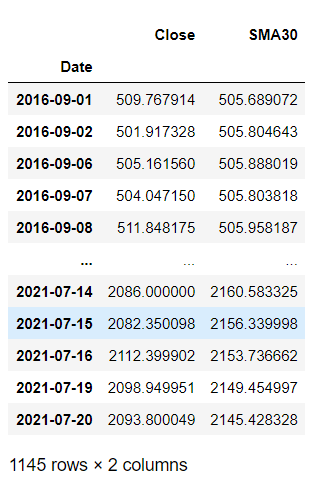## Python3

 `# plotting Close price and simple` `# moving average of 30 days using .plot() method` `reliance[[``'Close'``, ``'SMA30'``]].plot(label``=``'RELIANCE'``, ` `                                  ``figsize``=``(``16``, ``8``))`

Output: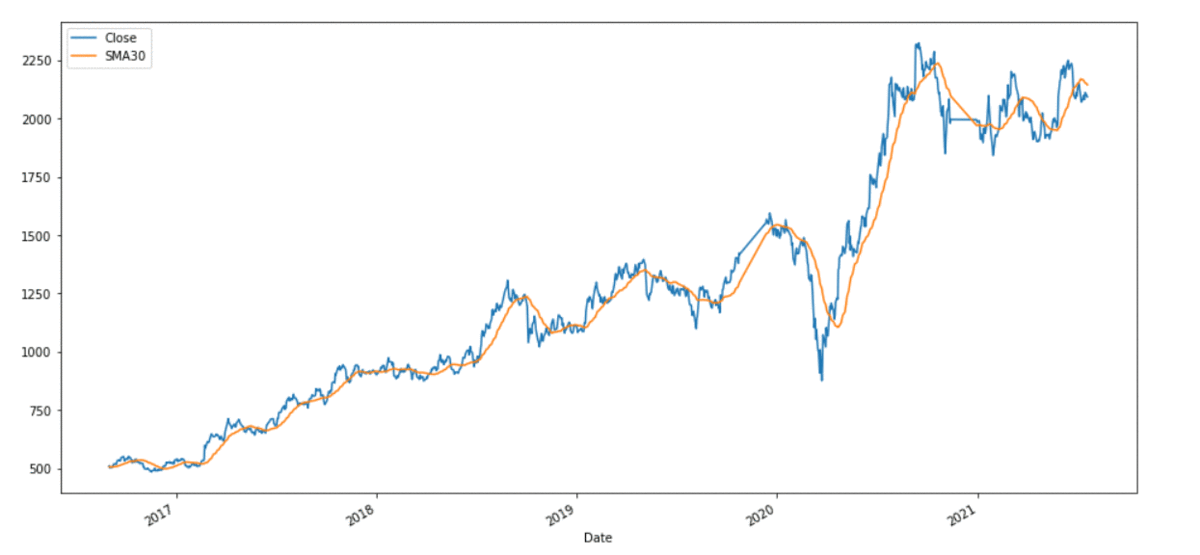## Cumulative Moving Average (CMA)

The Cumulative Moving Average is the mean of all the previous values up to the current value. CMA of dataPoints x1, x2 …..  at time t can be calculated as,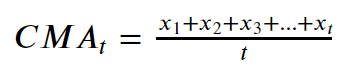While calculating CMA we don’t have any fixed size of the window. The size of the window keeps on increasing as time passes. In Python, we can calculate CMA using .expanding() method. Now we will see an example, to calculate CMA for a period of 30 days.

## Python3

 `# importing Libraries`   `# importing pandas as pd` `import` `pandas as pd`   `# importing numpy as np` `# for Mathematical calculations` `import` `numpy as np`   `# importing pyplot from matplotlib as plt` `# for plotting graphs` `import` `matplotlib.pyplot as plt` `plt.style.use(``'default'``)` `%``matplotlib inline`

### Step 2: Importing Data

To import data we will use pandas  .read_csv() function.

## Python3

 `# importing time-series data` `reliance ``=` `pd.read_csv(``'RELIANCE.NS.csv'``, ` `                       ``index_col``=``'Date'``,` `                       ``parse_dates``=``True``)`   `# Printing dataFrame` `reliance.head()`

### Step 3: Calculating Cumulative Moving Average

To calculate CMA in Python we will use dataframe.expanding() function. This method gives us the cumulative value of our aggregation function (mean in this case).

Syntax: DataFrame.expanding(min_periods=1, center=None, axis=0, method=’single’).mean()

Parameters:

• min_periods : int, default 1 . Least number of observations in a window required to have a value (otherwise result is NA).
• center : bool, default False . It is used to set the labels at the center of the window.
• axis : int or str, default 0
• method : str {‘single’, ‘table’}, default ‘single’

## Python3

 `# updating our dataFrame to have only` `# one column 'Close' as rest all columns ` `# are of no use for us at the moment` `# using .to_frame() to convert pandas series` `# into dataframe.` `reliance ``=` `reliance[``'Close'``].to_frame()`   `# calculating cumulative moving` `# average using .expanding().mean()` `reliance[``'CMA30'``] ``=` `reliance[``'Close'``].expanding().mean()`   `# printing Dataframe` `reliance`

Output: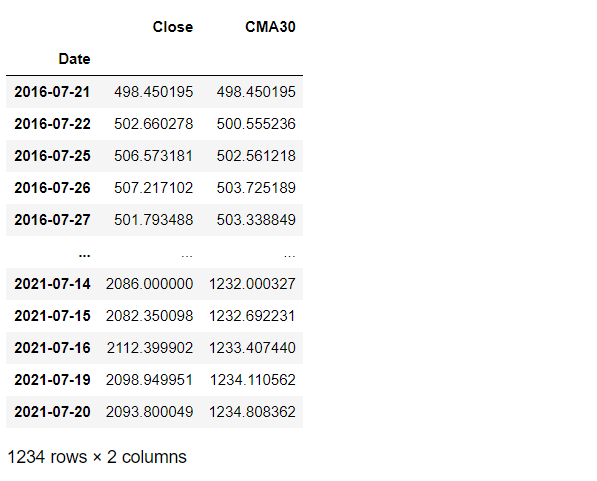## Python3

 `# plotting Close price and cumulative moving` `# average of 30 days using .plot() method` `reliance[[``'Close'``, ``'CMA30'``]].plot(label``=``'RELIANCE'``, ` `                                  ``figsize``=``(``16``, ``8``))`

Output: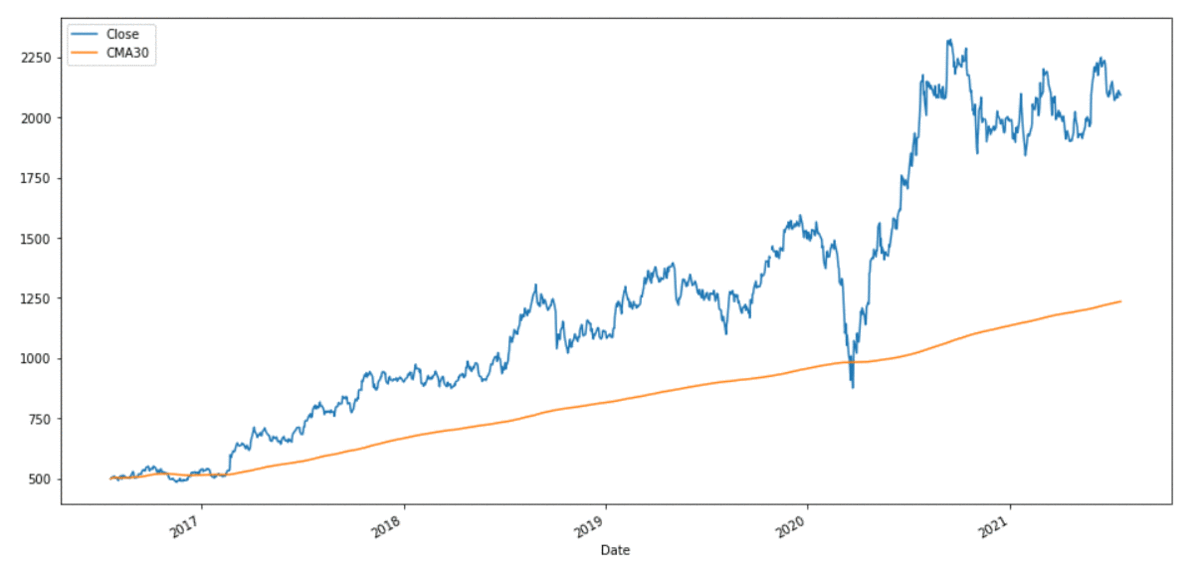## Exponential moving average (EMA):

Exponential moving average (EMA) tells us the weighted mean of the previous K data points. EMA places a greater weight and significance on the most recent data points. The formula to calculate EMA at the time period t is: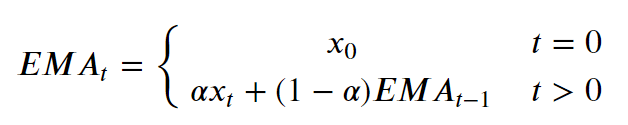where xt is the value of observation at time t & α is the smoothing factor. In Python, EMA is calculated using .ewm() method. We can pass span or window as a parameter to .ewm(span = ) method.

Now we will be looking at an example to calculate EMA for a period of 30 days.

## Python3

 `# importing Libraries`   `# importing pandas as pd` `import` `pandas as pd`   `# importing numpy as np` `# for Mathematical calculations` `import` `numpy as np`   `# importing pyplot from matplotlib as plt` `# for plotting graphs` `import` `matplotlib.pyplot as plt` `plt.style.use(``'default'``)` `%``matplotlib inline`

### Step 2: Importing Data

To import data we will use pandas  .read_csv() function.

## Python3

 `# importing time-series data` `reliance ``=` `pd.read_csv(``'RELIANCE.NS.csv'``,` `                       ``index_col``=``'Date'``,` `                       ``parse_dates``=``True``)`   `# Printing dataFrame` `reliance.head()`

Output:### Step 3: Calculating Exponential Moving Average

To calculate EMA in Python we use dataframe.ewm() function. It provides us exponentially weighted functions. We will be using .mean() function to calculate EMA.

Syntax: DataFrame.ewm(com=None, span=None, halflife=None, alpha=None, min_periods=0, adjust=True, ignore_na=False, axis=0, times=None).mean()

Parameters:

• com : float, optional . It is the decay in terms of centre of mass.
• span : float, optional . It is the decay in terms of span.
• halflife : float, str, timedelta, optional . It is the decay in terms of halflife.
• alpha : float, optional . It is the smoothing factor having value between 0 and 1 , 1 inclusive .
• min_periods : int, default 0. Least number of observations in a window required to have a value (otherwise result is NA).
• adjust : bool, default True . Divide by decaying adjustment factor in beginning periods to account for imbalance in relative weightings (viewing EWMA as a moving average)
• ignore_na : Ignore missing values when calculating weights; specify True to reproduce pre-0.15.0 behavior.
• axis : The axis to use. The value 0 identifies the rows, and 1 identifies the columns.

## Python3

 `# updating our dataFrame to have only` `# one column 'Close' as rest all columns` `# are of no use for us at the moment` `# using .to_frame() to convert pandas ` `# series into dataframe.` `reliance ``=` `reliance[``'Close'``].to_frame()`   `# calculating exponential moving average` `# using .ewm(span).mean() , with window size = 30` `reliance[``'EWMA30'``] ``=` `reliance[``'Close'``].ewm(span``=``30``).mean()`   `# printing Dataframe` `reliance`

Output: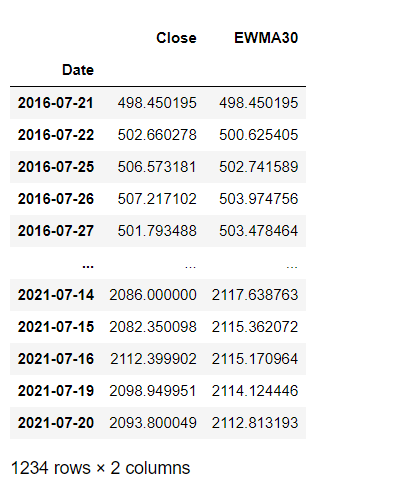## Python3

 `# plotting Close price and exponential ` `# moving averages of 30 days` `# using .plot() method` `reliance[[``'Close'``, ``'EWMA30'``]].plot(label``=``'RELIANCE'``,` `                                   ``figsize``=``(``16``, ``8``))`

Output: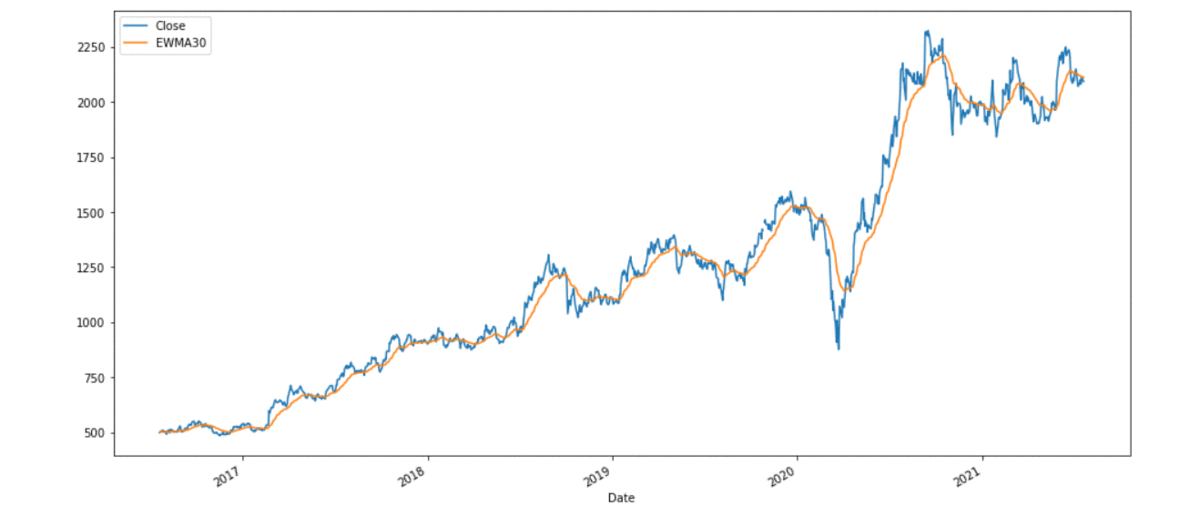Whether you're preparing for your first job interview or aiming to upskill in this ever-evolving tech landscape, GeeksforGeeks Courses are your key to success. We provide top-quality content at affordable prices, all geared towards accelerating your growth in a time-bound manner. Join the millions we've already empowered, and we're here to do the same for you. Don't miss out - check it out now!